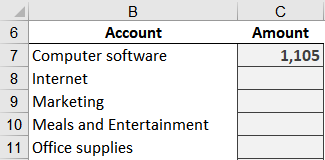# Battle of Heavyweights: SUMIFSThis is the second post in the Battle of Heavyweights series, where we are comparing VLOOKUP to SUMIFS in order to understand their key differences. Knowing these key differences will help us determine which to use in a given workbook. In the previous post, we were introduced to VLOOKUP. Now, it is time to meet the challenger: SUMIFS.

## SUMIFS

SUMIFS is a conditional summing function. That means it adds stuff up …. like SUM; but it is conditional … meaning it only includes selected rows.

Let’s get to an example.

Let’s say we need to compute the totals for a few selected accounts. We enter the account names into some cells and leave a column for Amount, like this:To figure out the amount values, we need to look through our transaction table and add up various rows. Here is a look at the the first several rows of the transactions table (Table1):To complete our report, we need to add up the transactions for each account. This is where SUMIFS will help.

Let’s think about the information SUMIFS will need to compute the total for each account. It will need to know which column in the data table contains the amount values. However, we don’t want the result to include all of the rows in that column. We only want to include some of the rows … that is, the rows that contain transactions for a specific account. So, it needs to know which column contains the account and which account to aggregate. Fortunately, the SUMIFS arguments are set up in exactly this order:

=SUMIFS(sum_range, criteria_range1, criteria1, …)

Where:

• sum_range is the column of numbers to add
• criteria_range1 is the column that contains the account names
• criteria1 is the specific account

Let’s give it a try. We write the following formula into C7:

=SUMIFS(Table1[Amount], Table1[Account], B7)

Where:

• Table1[Amount] is the column of numbers to add
• Table1[Account] is the column of account names
• B7 is the cell with our first account name

We hit Enter, and bam:We push the formula down, and bam:Yay … it works!

Here are a few notes:

• SUMIFS handles multiple conditions, and each condition is defined with a pair of arguments (note: SUMIF handles a single condition; SUMIFS handles multiple conditions).
• SUMIFS uses “and” logic, meaning all conditions must be true for that row to be included in the sum. If you need to use “or” logic instead, check out this post.
• We could prepare the specific report used in this post with a PivotTable. However, SUMIFS enables us to present summary data in ways that PivotTables aren’t designed to, for example, in financial statements. In fact, check out this post if you want to create a balance sheet with SUMIFS.

## Conclusion

So, to recap, SUMIFS is a conditional summing function. It adds a column of numbers, but, only includes certain rows. It only includes the rows that meet the specified condition.

Now that we have met our two competitors VLOOKUP and SUMIFS … the battle begins in the next post!

Sample file: IntroducingSUMIFS.xlsx

Get a quick email notice when a new Excel article is available

• This field is for validation purposes and should be left unchanged.1.Lori

While SUMIFS is indeed useful, I use VLOOKUP more than all other functions combined! I literally use it every workday. I preach to anyone who’ll listen that if you use Excel, learn VLOOKUP.

1.Jeff Lenning Post author

I love VLOOKUP too … and 100% agree it is a critical, must-know function 🙂

2.Carl Walton

1.Jeff Lenning Post author
3.Carl Walton